# Review — Learning Deep Transformer Models for Machine Translation

## Pre-Norm Transformer, Layer Normalization First

Learning Deep Transformer Models for Machine Translation
Pre-Norm Transformer, by Northeastern University, NiuTrans Co., Ltd., Kingsoft AI Lab, and University of Macau
2019 ACL, Over 200 Citations (Sik-Ho Tsang @ Medium)
Natural Language Processing, NLP, Machine Translation, Transformer

• Two novel techniques are proposed:
1. A proper use of layer normalization is proposed, called pre-norm Transformer, and;
2. A novel way of passing the combination of previous layers to the next.

# Outline

1. Pre-Norm Transformer
2. Dynamic Linear Combination of Layers (DLCL)
3. Experimental Results

# 1. Pre-Norm Transformer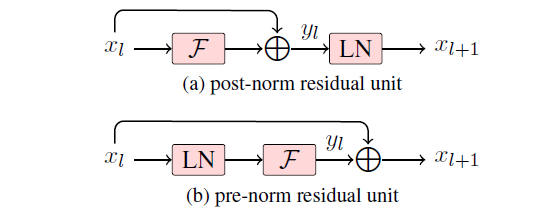(a) Original Post-Norm Transformer (b) Proposed Pre-Norm Transformer

## 1.1. Original Post-Norm Transformer

• On the encoder side, there are a number of identical stacked layers. Each of them is composed of a self-attention sub-layer and a feed-forward sub-layer.
• The attention model used in Transformer is multi-head attention, and its output is fed into a fully connected feed-forward network.
• For Transformer, it is not easy to train stacked layers, residual connections and layer normalization are adopted:
• where xl and xl+1 are the input and output of the l-th sub-layer, and yl is the intermediate output followed by the post-processing function f().
• Layer normalization is adopted to reduce the variance of sub-layer output, and it is placed after the element-wise residual addition:
• It can be seen as a post-processing step of the output.

## 1.2. Proposed Pre-Norm Transformer

• The above equation regards layer normalization as a part of the sub-layer, and does nothing for post-processing of the residual connection.

## 1.3. Gradients of Post-Norm

• A stack of L sub-layers are used as an example. Let E be the loss used to measure how many errors occur in system prediction, and xL be the output of the topmost sub-layer.
• For post-norm Transformer, given a sub-layer l, the differential of E with respect to xl can be computed by the chain rule, and we have:

The above equation is inefficient for passing gradients back because the residual connection is not a bypass of the layer normalization unit.

## 1.4. Gradients of Pre-Norm

• Likewise, we have the gradient for pre-norm:

Obviously, the above equation for pre-norm establishes a direct way to pass error gradient ∂E/∂xL from top to bottom. Its merit lies in that the number of product items on the right side does not depend on the depth of the stack.

# 2. Dynamic Linear Combination of Layers (DLCL)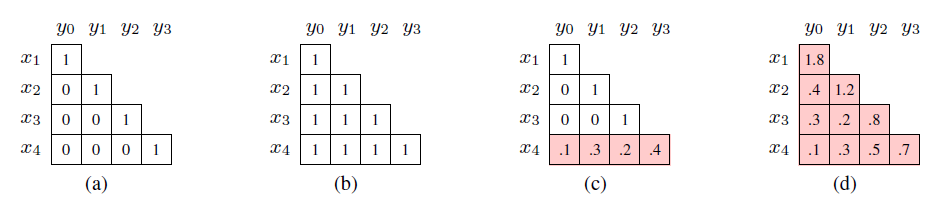Connection weights for 3-layer encoder: (a) residual connection (b) dense residual connection (c) multi-layer representation fusion (d) proposed DLCL

DLCL is proposed to make direct links with all previous layers and offers efficient access to lower-level representations in a deep stack.

• Let {y0, …, yl} be the output of layers 0~l. The input of layer l+1 is defined to be:
• where G() is a linear function that merges previously generated values {y0, …, yl} into a new value.
• For pre-norm Transformer, G() is defined as:

where W(l+1)k is a learnable scalar and weights each incoming layer in a linear manner. The above equation provides a way to learn preference of layers in different levels of the stack.

• For post-norm, G() can be redefined as:

# 3. Experimental Results

## 3.1. SOTA Comparison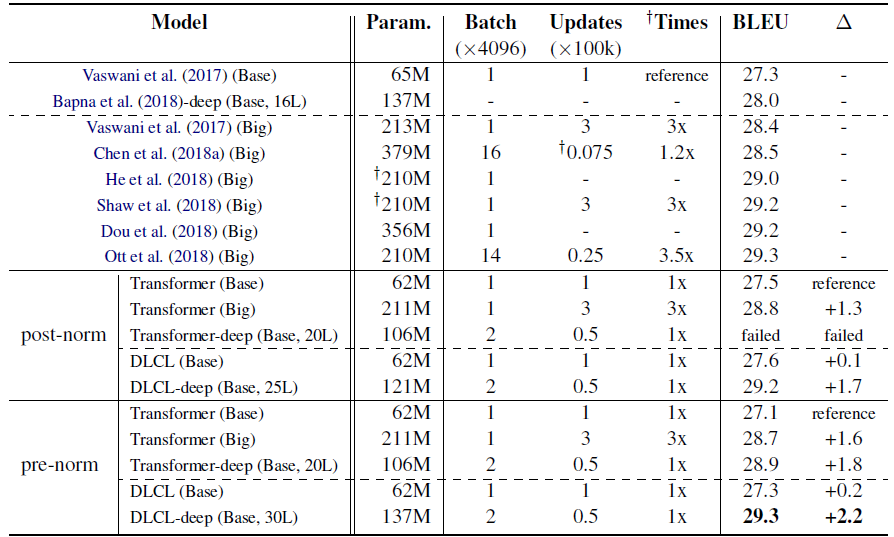BLEU scores [%] on English-German translation
• When increasing the encoder depth, e.g. L=20, the vanilla Transformer failed to train. On the contrary, post-norm DLCL solves this issue and achieves the best result when L=25.

Pre-norm slightly underperforms the post-norm counterpart in shallow networks, pre-norm Transformer benefits more from the increase in encoder depth.

• Pre-norm is easier to optimize than post-norm in deep networks. Beyond that, a 30-layer encoder is successfully trained, resulting in a further improvement of 0.4 BLEU points. This is 0.6 BLEU points higher than the pre-norm Transformer-Big.

Although the best score of 29.3 is the same as Ott et al. (2018), the proposed approach only requires 3.5× fewer training epochs than theirs.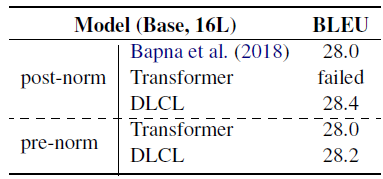Compare with Bapna et al. (2018) on WMT’16 English-German translation under a 16-layer encoder
• DLCL in both post-norm and pre-norm cases outperform Transparent Atenttion (TA) by Bapna et al. (2018).

## 3.2. Zh-En-Small Task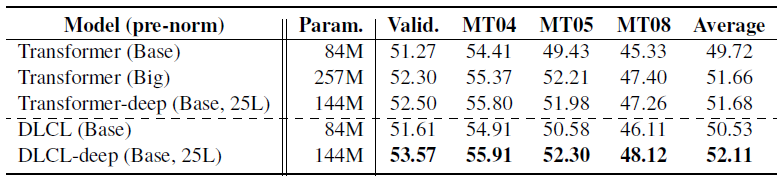BLEU scores [%] on NIST’12 Chinese-English translation
• Firstly DLCL is superior to the baseline when the network’s depth is shallow. Interestingly, both Transformer and DLCL achieve the best results when a 25-layer encoder is used.

## 3.3. Zh-En-Large Task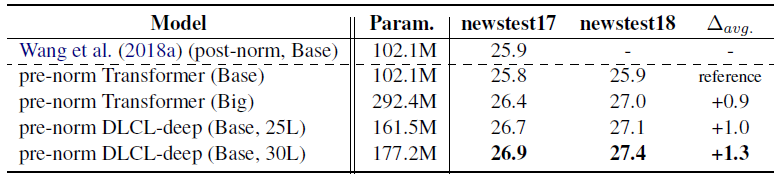BLEU scores [%] on WMT’18 Chinese-English translation
• The 25-layer pre-norm DLCL slightly surpassed Transformer-Big, and the superiority is bigger when using a 30-layer encoder.

## 3.4. Effect of Encoder Depth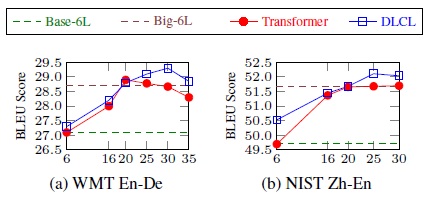BLEU scores [%] against the encoder depth for pre-norm Transformer and pre-norm DLCL on English-German and Chinese-English tasks.
• Remarkably, when the encoder depth reaches 20, both of the two deep models can achieve comparable performance to Transformer-Big.

Pre-norm Transformer degenerates earlier and is less robust than DLCL when the depth is beyond 20.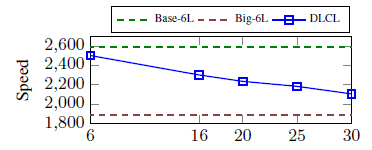GPU generation speed (target tokens/sec.) against the depth of encoder for pre-norm DLCL on English-German task (batch size = 32, beam size = 4).

The proposed system with a 30-layer encoder is still faster than Transformer-Big.

## 3.5. Effect of Decoder Depth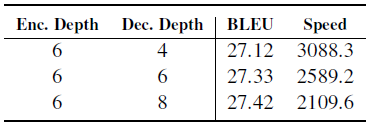Tokenized BLEU scores [%] and GPU generation speed (target tokens per second) in pre-norm Transformer (Base) on the test set of WMT English-German (batch size = 32, beam size = 4).

Different from encoder, increasing the depth of decoder only yields a slight BLEU improvement.

## 3.6. Effect of DLCL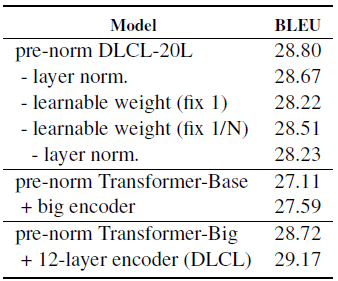Ablation results by tokenized BLEU [%] on the test set of WMT English-German translation
• Replacing learnable weights with constant weights: All-One (Wij=1) and Average (Wij=1/(i+1)) consistently hurt performance.

Making the weights learnable is important.

## 3.7. Weight Visualization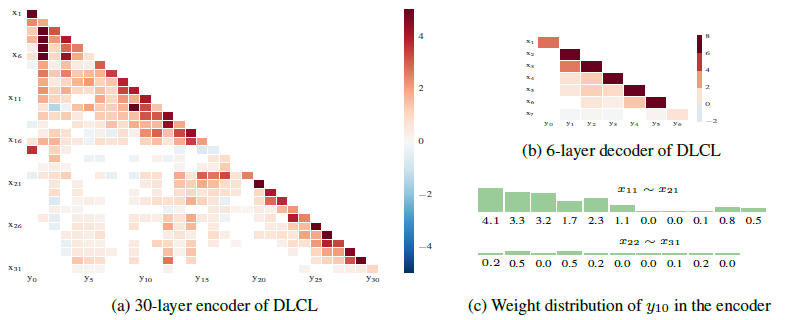A visualization example of learned weights in 30-layer pre-norm DLCL model
• The connections in the early layers are dense, but become sparse as the depth increases.
• Most of the large weight values concentrate on the right of the matrix, which indicates that the impact of the incoming layer is usually related to the distance between the outgoing layer, but the contribution to successive layers changes dynamically (one column).

## Reference

[2019 ACL] [Pre-Norm Transformer]
Learning Deep Transformer Models for Machine Translation

## Machine Translation

2014 [Seq2Seq] [RNN Encoder-Decoder] 2015 [Attention Decoder/RNNSearch] 2016 [GNMT] [ByteNet] [Deep-ED & Deep-Att] 2017 [ConvS2S] [Transformer] [MoE] [GMNMT] [CoVe] 2018 [Shaw NAACL’18] 2019 [AdaNorm] [GPT-2] [Pre-Norm Transformer] 2020 [Batch Augment, BA] [GPT-3] [T5] 2021 [ResMLP]

--

--